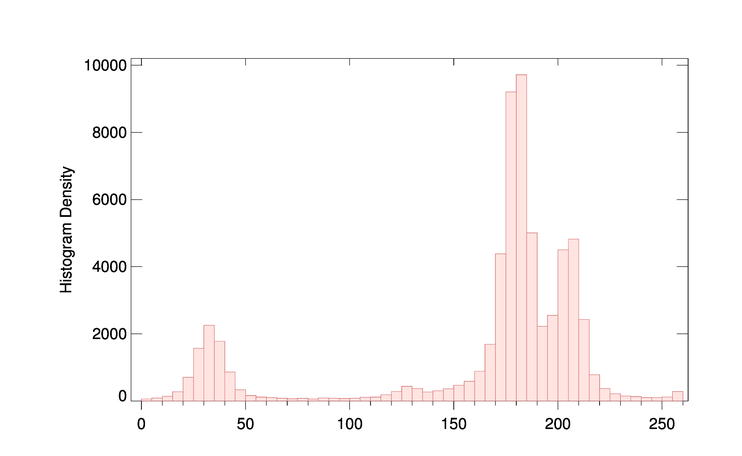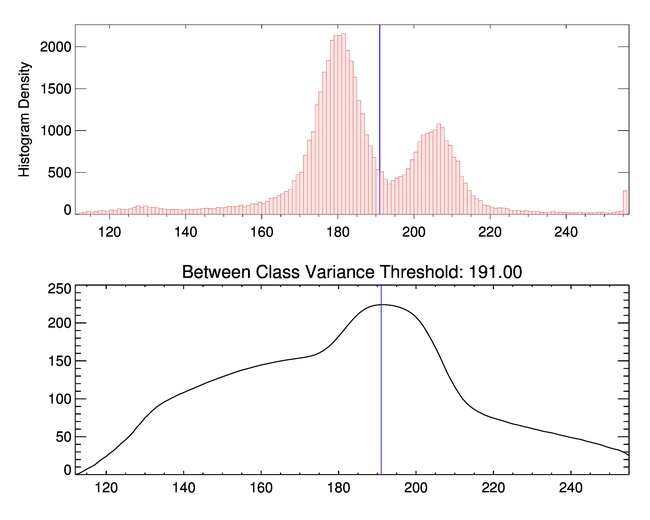Separating Bimodal Distributions with Otsu ThresholdQUESTION: I have a bimodal population of pixels in an image, and I would like to automatically find a threshold that can separate the two populations. Is there anything like this in IDL?ANSWER: Yes, you can use the cgOtsu_Threshold program to find the statistically optimal threshold to separate a bimodal population of pixels. This program uses the "faster algorithm" approach on this web page to calculate the Otsu Threshold, which is based on the image's histogram. The method involves maximizing the inter-class variances of the two separate pixel populations.

Consider the mineral micrograph image in the IDL examples directory. Here is a histogram of its pixel values.

image = cgDemoData(25)
cgHistoplot, image, /Fill

You see the result in the figure below. There is a population of pixels in the 0 to 50 range, and another population with values above 150.This image has two clearly separate pixel populations.

To find the optimal threshold, you can type this.

bimodal_threshold = cgOtsu_Threshold(image, /PlotIt)

As you can see from the figure below, the optimal threshold to separate these two pixel populations is 112.The optimal threshold to separate these pixel populations is 112.

The pixels with values greater than 112 also seem to have a bimodal distribution. You can find an optimal for separating those two populations by using cgOtsu_Threshold again, this image by seeing the Min keyword to the previous threshold.

threshold = cgOtsu_Threshold(image, /PlotIt, MIN=bimodal_threshold)

In this case, the new threshold is 191, as shown in the figure below.The optimal threshold to separate these pixel populations above 112 is 191.